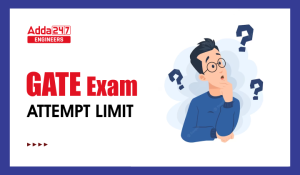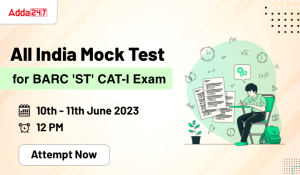Engineering Jobs   »   Civil Engineering quizs   »   UPSSSC JE QUIZ

# UPSSSC-JE’21 CE: Daily Practice Quiz. 05-October-2021

Know your strengths and practice your concepts with this quiz on UPSSSC-JE Recruitment 2021. This quiz for UPSSSC-JE Recruitment 2021 is designed specially according to UPSSSC-JE Syllabus 2021.

Quiz: Civil Engineering
Exam: UPSSSC-JE
Topic: MISCELLANEOUS

Each question carries 1 mark
Negative marking: 1/4 mark
Time: 8 Minutes

Q1. When H is the flight height, R is the appropriate radial measure and d is the relief displacement the vertical height of an object appearing on an aerial photograph is
(a) dH/R
(b) R/dH
(c) RH/d
(d) H/dR

Q2. A rainfall with an intensity of 5 mm/hr is classified as
(a) Trace
(b) Light rain
(c) Moderate rain
(d) Heavy rain

Q3. Which of the following equations is used in hydrologic flood routing method?
(a) Lacey’s equation
(b) Equation of motion
(c) Energy equation
(d) Continuity equation

Q4. In a linear reservoir :
(a) outflow rate varies linearly with storage
(b) volume varies with elevation
(c) storage varies linearly with time
(d) storage varies linearly with inflow rate

Q5. Chlorine usage in the treatment of 25000 m³/day of water has been 9 kg/day. The residual chlorine after 10 minutes contact is 0.2 mg/l. The chlorine demand of water would be nearly
(a) 0.12 mg/l
(b) 0.16 mg/l
(c) 0.22 mg/l
(d) 0.28 mg/l

Q6. The unit in which both sedimentation and digestion processes of sludge take place simultaneously is called:
(a) Skimming tank
(b) Detritus tank
(c) Imhoff tank
(d) Digestion tank

SOLUTION

S1. Ans.(a)
Sol. Relief displacement is given as-
▭(d=Rh/H)
Vertical height ▭((h)=dH/R)
Where d = relief displacement
H = flying height

S2. Ans.(c)
Sol.

 Intensity of Rainfall Rain type 0-2.5 mm/Hr. Light Rain 2.5-7.5 mm/Hr. Moderate rain >7.5 mm/Hr. Heavy rain

S3. Ans.(d)
Sol. In hydrologic flood routing, continuity equation is used.
Q=A_1 V_1=A_2 V_2

S4. Ans.(a)
Sol. in a linear reservoir, outflow rate varies linearly with storage.

S5. Ans.(b)
Sol. Given,
Quantity of water treated = 25000 m³/day
Chlorine use = 9 kg/day
Chlorine dose = (Chlorine used)/(Quantity of water treated)
=(9 kg/day)/(25000 m^3/day)
=(9×10^6 mg/day)/(25000×10^3 l/day)
= 0.36 mg/l
Chlorine demand = Chlorine dose – Residual chlorine
= 0.36 – 0.2
= 0.16 mg/l

S6. Ans.(c)
Sol. An Imhoff tank is an improvement over septic tank in which both sedimentation and digestion process of sludge takes place.

Sharing is caring!

•GATE Result 2023 Out, Download Result, C...
•GATE 2023 Admit Card Out, Download Link ...
•JSSC JE Recruitment 2023 Out, Apply Onli...
•GATE Exam Attempt Limit, Check Here
•How to Prepare for GATE 2024 in 6 Months
•All India Mock Test For DDA JE Exam 2023...
•All India Mock Test for BARC 'ST' CAT-I ...
•DDA JE Recruitment 2023 Out, Apply Onlin...